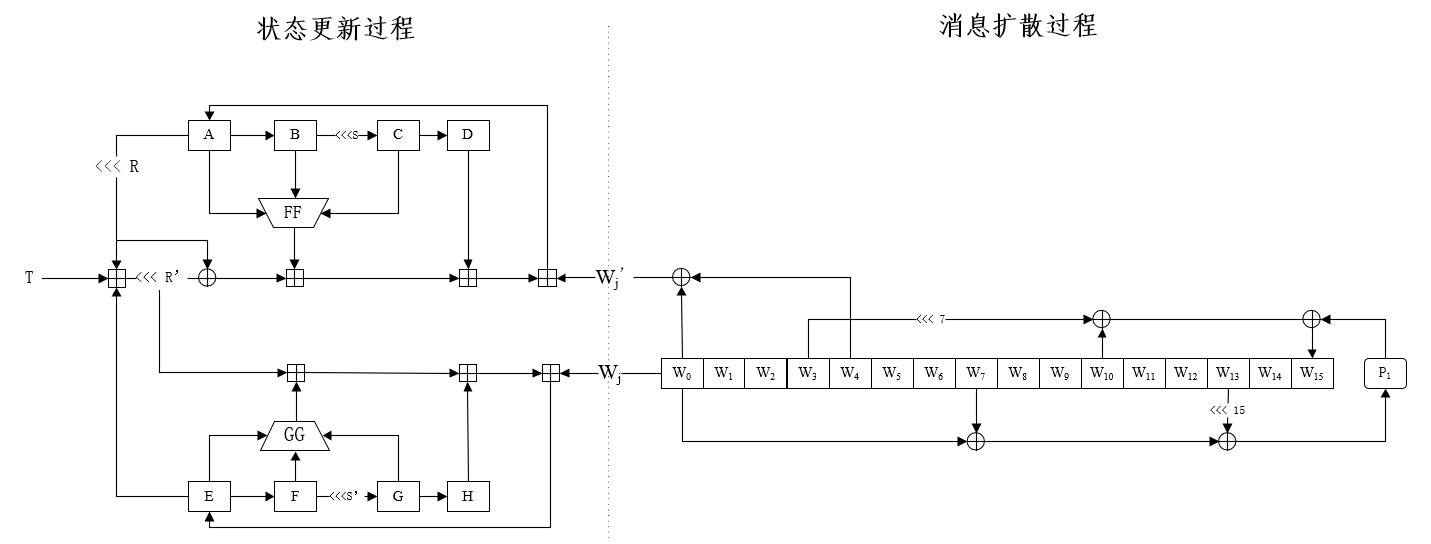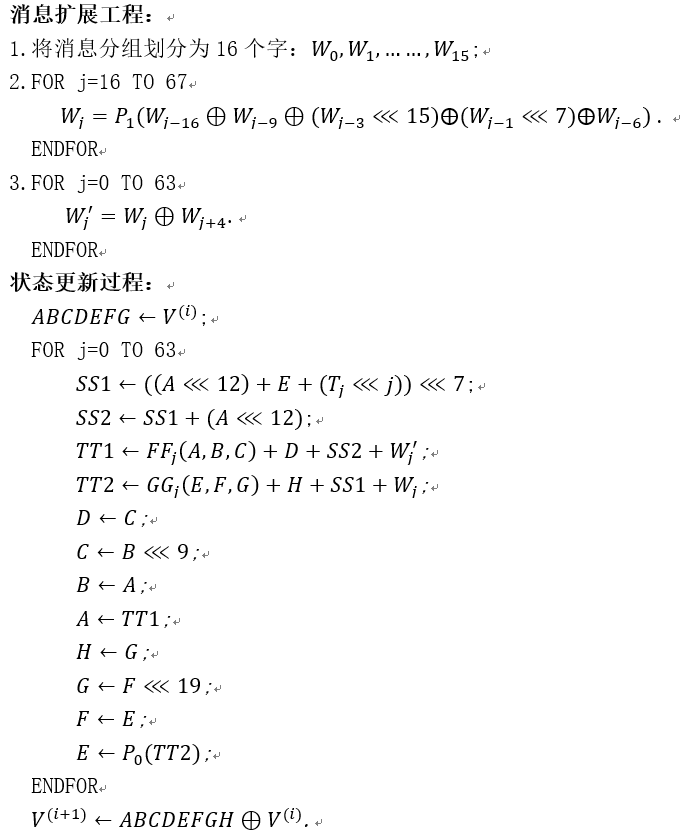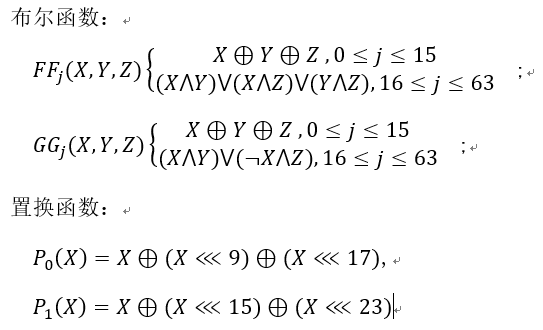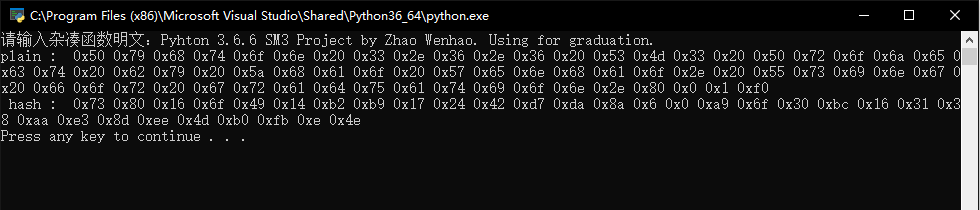# SM3杂凑算法实现——第三部分

## 一、SM3 密码概述

我们首先把需要用到的算法呈现出来，最后我们再考虑如何集合为一个库的方法，这一部分我们就开始编写一个新的算法：国家商用密码标准SM3密码算法。

首先要明白SM3是一个什么样的东西：单向加密算法。也可以称之为密码哈希算法、杂凑算法、摘要算法，都可以指这类算法。顾名思义，这类算法只能加密不能解密，所以不是为了直接保护数据的秘密性而存在的，不是让使用者解密这串密文得到原文而使用的。这类算法一般用于保护数据明文的完整性，抗篡改而产生。只要输入的是同样的明文，那么输出的密文（杂凑值/摘要值/哈希结果）就是一样的，而找到一个字符串与预期字符串的输出结果是一样的，这目前在理论上不可实现，也就是说在目前的技术前提下，还不能做到篡改原文还能保持摘要值不受影响的事情。这类算法比较出名的比如MD5，SHA-1代表的系列算法，但其中部分算法如MD5已经被证实并不安全，所以在实际使用过程中一定要使用当前核准的优秀加密算法！

密码杂凑算法在现代密码学中起着重要的作用，它将任意长度的消息压缩成固定长度的摘要。它是密码学3大基础算法之一（加密算法、数字签名算法和杂凑算法），用于数据的完整性校验、身份认证、数字签名、密钥推导、消息认证码和随机比特生成器等。

2012年，国家商用密码管理办公室发布了SM3密码杂凑算法为密码行业标准。2016年，国家标准化委员会公布了SM3密码杂凑算法为国家标准。目前SM3已经提交ISO国际标准化组织，进入DIS阶段。

## 二、SM3 详细设计

如所有的密码杂凑算法，SM3没有密钥这个概念，输入数据长度为任意，输出长度为32字节（256-bit）下面我们按步骤一点点看：

### 1.初始化常量

废话不多说，简单的定义：

IV = "\x73\x80\x16\x6f\x49\x14\xb2\xb9\x17\x24\x42\xd7\xda\x8a\x06\x00\xa9\x6f\x30\xbc\x16\x31\x38\xaa\xe3\x8d\xee\x4d\xb0\xfb\x0e\x4e"
T0_15 = "\x79\xcc\x45\x19"
T16_63 = "\x7a\x87\x9d\x8a"


其中T0_15表示CF模块中轮数0<=j<16时取用的值，T16_63表示轮数16<=j<64时取用的T值。IV为初始状态常量。

### 2.明文消息填充

对长度为l(l<2^64)比特的消息m，首先将比特'1'填充至消息末尾，再添加k个'0'，其中k满足l+k+1===448 mod512,取最小非负整数。再添加一个64比特字符串，该比特串是长度l的二进制表示。这样，我们就把明文填充至512比特的倍数长度。

看不太明白？代码见！

### 3.迭代压缩结构

填充后的消息M就可以按照512bit进行分组啦！依次分为n个消息组，分别与初始常量IV按以下方式进行迭代：

FOR i=0 TO (n-1):
V[i+1] = CF( V[i] , B[i] );
ENDFOR


其中CF为压缩函数，V = IV，B为填充后的消息分组，迭代的输出结果就是最后依次循环中被赋值的变量V[n]。

### 4.压缩函数

咱先不说那些复杂的公式，先看图理解一下，这个部分的结构比较庞大：右半部分与序列密码中的部分很是相似，可以将原来的数据进行充分的混合，随着寄存器不停地进动，左端的信息会进入状态更新区内，与数据V进行混合运算，再次充分混淆。这样一来，每部分输出的信息V都是本段数据和之前所有数据共同运算的结果，可以代表这之前的数据是完整无修改的。公示详述如下：将最后一轮产生的V进行输出，即得到杂凑值运算结果！

这里面显然有几个地方还没有定义，往下看：

### 5.布尔函数和置换函数

在CF压缩函数中涉及到的布尔函数和置换函数的详细定义描述为公式如下：如此设计的布尔函数和置换函数让每个部分的数据都参与运算且运算效率极高，配合CF结构保证了运算的非线性特性明显。

至此，整个算法的介绍到此为止。

## 三、Python语言程序实现

# 数字格式转化
def int2str( num ) :
out = ""
out = out + chr( num//65536 ) + chr( (num%65536)//256 ) + chr( num%256 )
return out

def str2hex( string ):
out = ""
for i in range ( 0 , len(string) ):
out = out + " " + hex(ord( string[i] ))
return out

# 字符串异或运算，后面分别是字符串与、或、非运算
def strxor( message , key , len ):
out = ""
for i in range ( 0 , len ):
ch = ord(message[i]) ^ ord(key[i])
out = out + chr(ch)
return out

def strand( message , key , len ):
out = ""
for i in range ( 0 , len ):
ch = ord(message[i]) & ord(key[i])
out = out + chr(ch)
return out

def stror( message , key , len ):
out = ""
for i in range ( 0 , len ):
ch = ord(message[i]) | ord(key[i])
out = out + chr(ch)
return out

def strnot( string , len ) :
out = ""
for i in range ( 0 , len ) :
ch = ~ord(string[i])
out = out + chr(ch)
return out

# 字符串按比特向左移位
def strldc( string , bit ):
byte = bit // 8
bit = bit % 8
out = ""
if bit == 0 :
out = string[byte:] + string[:byte]
else :
reg = string[byte:] + string[:byte+1]
for i in range (0,len(reg)-1):
out = out + chr(((ord(reg[i])*(2**bit))+(ord(reg[i+1])//(2**(8-bit))))%256)
out = out[:len(string)]
return out

# 4字节模加运算
def stradd_4( str1 , str2 ) :
out = ""
num1 = ord(str1)*16777216+ord(str1)*65536+ord(str1)*256+ord(str1)
num2 = ord(str2)*16777216+ord(str2)*65536+ord(str2)*256+ord(str2)
return out

def functionFF( A , B , C , j ) :
if j < 16 :
FF = strxor( A , strxor( B , C , 4 ) , 4 )
if j > 15 :
FF = stror( stror( strand(A,B,4) , strand(A,C,4) , 4 ) , strand(B,C,4) , 4)
return FF

def functionGG( A , B , C , j ) :
if j<16 :
GG = strxor( A , strxor( B , C , 4 ) , 4 )
if j > 15 :
GG = stror( strand(A,B,4) , strand(strnot(A,4),C,4) , 4 )
return GG

def functionP( string , mode ) :
out = ''
if mode == 0 :
out = strxor( string , strxor( strldc(string,9) , strldc(string,17) , 4 ) , 4 )
if mode == 1 :
out = strxor( string , strxor( strldc(string,15) , strldc(string,23) , 4 ) , 4 )
return out

def functionCF( V , B ) :
for i in range (0,68) :
# 消息扩展过程
P = strxor( strxor( B[0:4] , B[28:32] , 4 ) , strldc( B[42:46] , 15 ) , 4)
Badd = strxor( P , strxor( B[40:44] , strldc(B[12:16],7) , 4 ) , 4 )
out1 = strxor( B[0:4] , B[16:20] , 4 )
out = B[0:4]
# 状态更新过程
if i < 64 :
if i < 16 :
SS1 = strldc( stradd_4( strldc( V[0:4] , 12 ) , stradd_4( V[16:20] , strldc(T0_15,i%32) ) ) , 7 )
else :
SS1 = strldc( stradd_4( strldc( V[0:4] , 12 ) , stradd_4( V[16:20] , strldc(T16_63,i%32) ) ) , 7 )
SS2 = strxor( SS1 , strldc( V[0:4] , 12 ) , 4 )
TT1 = stradd_4( stradd_4( functionFF( V[0:4] , V[4:8] , V[8:12] , i ) , V[12:16] ) , stradd_4( SS2 , out1 ) )
TT2 = stradd_4( stradd_4( functionGG( V[16:20] , V[20:24] , V[24:28] , i ) , V[28:32] ) , stradd_4( SS1 , out ) )
V = strxor( V , TT1 + V[0:4] + strldc(V[4:8],9) + V[8:12] + functionP(TT2,0) + V[16:20] + strldc(V[20:24],19) + V[24:28] , 32 )
return V

IV = "\x73\x80\x16\x6f\x49\x14\xb2\xb9\x17\x24\x42\xd7\xda\x8a\x06\x00\xa9\x6f\x30\xbc\x16\x31\x38\xaa\xe3\x8d\xee\x4d\xb0\xfb\x0e\x4e"
T0_15 = "\x79\xcc\x45\x19"
T16_63 = "\x7a\x87\x9d\x8a"
plain = input( "请输入杂凑函数明文：" )
l = int2str( len(plain)*8 )
plain = plain + "\x80"
k = 56 - (len(plain)%64) - 1
plain = plain + "\x00"*k
plain = plain + l
print( "plain :" , str2hex(plain) )
for i in range ( 0 , len(plain)//64-1 ) :
IV = functionCF( IV , plain[64*l:64*l+64] )
hash_value = IV
print( " hash :" , str2hex(hash_value) )


下面附上程序运行的效果图：由于SM3的功能不具备多样化特征，且代码长度短，这里暂不呈现SM3类的封装和使用过程，故代码方面的展示到此为止。

## 四、实现难点

杂凑算法一直以来没有尝试去实现过，也就是说这是作者第一次尝试去实现一个密码杂凑算法，刚开始做的时候压力还是很大的，但是随着算法步骤的一点点解读，才发现其中的内部结构并不复杂，都是由计算机基本运算组成的，但读懂文字解释的步骤还是相当有难度的。

论文依据：SM3密码杂凑算法——王小云、于红波。更多关于SM3密码的分析与设计细节详见论文。

posted @ 2019-02-18 10:08  囚月  阅读(4302)  评论(2编辑  收藏  举报